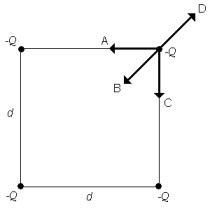# Problem: Four point charges of equal magnitude and sign are arranged on the corners of the square of sidedas shown in Fig. 21-5. Which of the arrows shown represents the net force acting on the charge at the upper right hand corner of the square?a. Ab. Bc. Cd. De. None of the above

###### FREE Expert Solution

This is a conceptual problem dealing with forces between charges.

83% (173 ratings)###### Problem Details

Four point charges of equal magnitude and sign are arranged on the corners of the square of sidedas shown in Fig. 21-5. Which of the arrows shown represents the net force acting on the charge at the upper right hand corner of the square?a. A

b. B

c. C

d. D

e. None of the above

Frequently Asked Questions

What scientific concept do you need to know in order to solve this problem?

Our tutors have indicated that to solve this problem you will need to apply the Coulomb's Law (Electric Force) concept. You can view video lessons to learn Coulomb's Law (Electric Force). Or if you need more Coulomb's Law (Electric Force) practice, you can also practice Coulomb's Law (Electric Force) practice problems.